# COMP7801 Topic 4 Top-k

## Background

### Multidimensional Data

• Flat relational tables
• Multimedia feature vectors
• Data warehouse data
• Spatial data
• Text documents

### Attribute Types

• Attributes of multidimensional tuples may have variable types
• Ordinal (e.g., age, salary)
• Nominal categorical values (e.g., color, religion)
• Binary (e.g., gender, owns_property)
• Basic queries: range, NN, similarity

### Basic Queries

• (Range) selection query
• Returns the records that qualify a (multidimensional) range predicate
• Example:
• Return the employees of age between 45 and 50 and salary above \$100,000
• Distance (similarity) query
• Returns the records that are within a distance from a reference record.
• Example:
• Find images with feature vectors of Euclidean distance at most ε with the feature vector of a given image
• Nearest neighbor (similarity) query
• Replaces distance bound by ranking predicate

## Top-k Search Methods

• Rank aggregation
• Index-based methods

### Top-k Query

• Given a set of objects (e.g., relational tuples),
• Returns the k objects with the highest combined score, based on an aggregate function f.
• Example:
• Relational table containing information about restaurants, with attributes(e.g. price, quality, location)
• f: sum(-price, quality, -dist(location,my_hotel))‏
• attribute value ranges are usually normalized
• E.g., all values in a (0,1) range
• otherwise some attribute may be favored in f

### Top-k Query Variants

• Apply on single table, or ranked lists of tuples ordered by individual attributes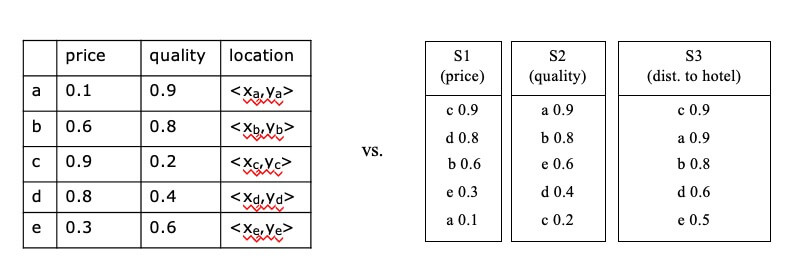• Ranked inputs in the same or different servers (centralized or distributed data)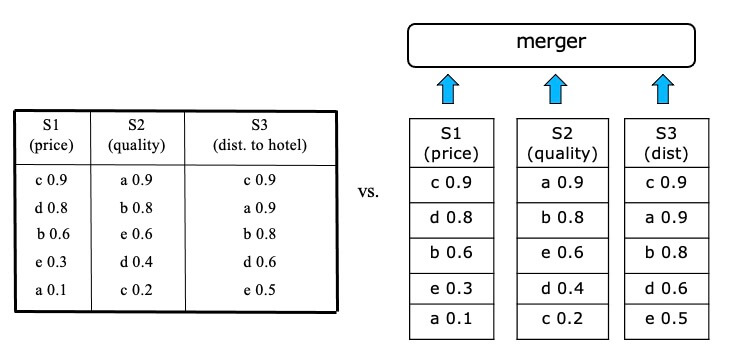• Standalone query or operator in a more complex query plan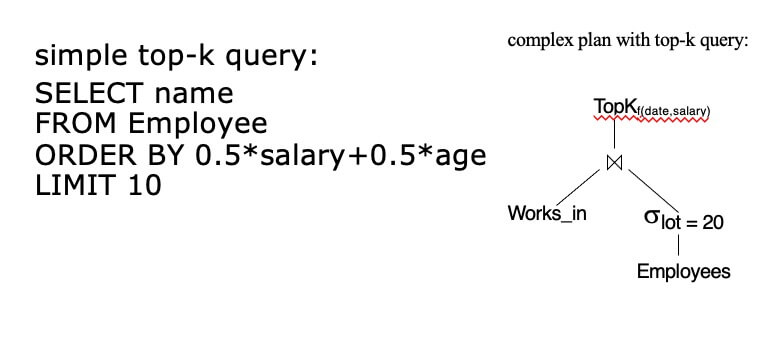• Incremental retrieval of objects with highest scores (k is not predefined)

• Top-k joins

``````SELECT h.id, s.id
FROM House h School s
WHERE h.location=s.location
ORDER BY h.price + 10 ∗ s.tuition
LIMIT 5
``````
• Probabilistic/approximate top-k retrieval

• Random and/or sorted accesses at ranked inputs

### Top-k Query Evaluation

• Most solutions assume distributive, monotone aggregate functions (e.g. f=sum)

• distributive: f(x,y,z,w)= f(f(x,y),f(z,w))
• e.g., A+B+C+D = (A+B) + (C+D)
• monotone: if x<y and z<w, then f(x,z)<f(y,w)
• Solutions based on 1-D ordering and merging sorted lists (rank aggregation)

• Solutions based on multidimensional indexing

## Rank Aggregation

• Solutions based on 1-D ordering and merging sorted lists (rank aggregation)
• Assume that there is a total ranking of the objects for each attribute that can be used in top-k queries
• These sorted inputs can be accessed sequentially and/or by random accesses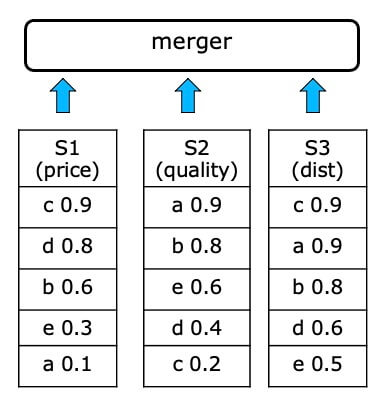• can be applied on any subset of inputs (arbitrary subspace)
• appropriate for distributed data
• appropriate for top-k joins
• easy to understand and implement
• Drawbacks:
• slower than index-based methods
• require inputs to be sorted

### TA: Threshold Algorithm

#### Introduction

• Iteratively retrieves objects and their atomic scores from the ranked inputs in a round-robin fashion.
• For each encountered object x, perform random accesses to the inputs where x has not been seen.
• Maintain top-k objects seen so far.
• T = f(\$l_1\$, . . . , \$l_m\$) is the score derived when applying the aggregation function to the last atomic scores seen at each input. If the score of the k-th object is no smaller than T, terminate.

#### Example of TA(k=1,f=sum)

• STEP 1
• top-1 is c, with score 2.0
• T=sum(0.9,0.9,0.9)=2.7
• T>top-1, we proceed to another round of accesses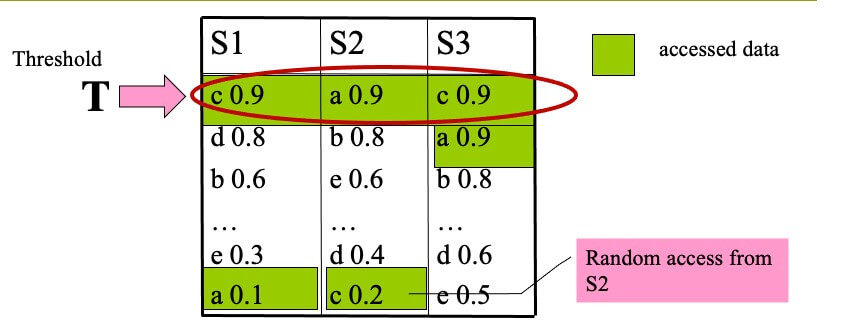• STEP 2
• top-1 is b, with score 2.2
• T=sum(0.8,0.8,0.9)=2.5
• T>top-1, we proceed to another round of accesses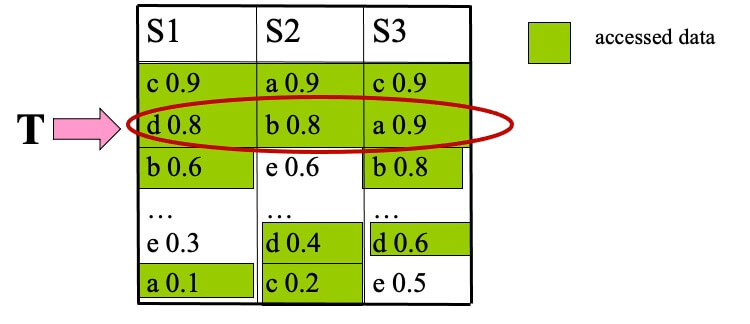• STEP 3
• top-1 is b, with score 2.2
• T=sum(0.6,0.6,0.8)=2.0
• T≤top-1, terminate and output (b,2.2)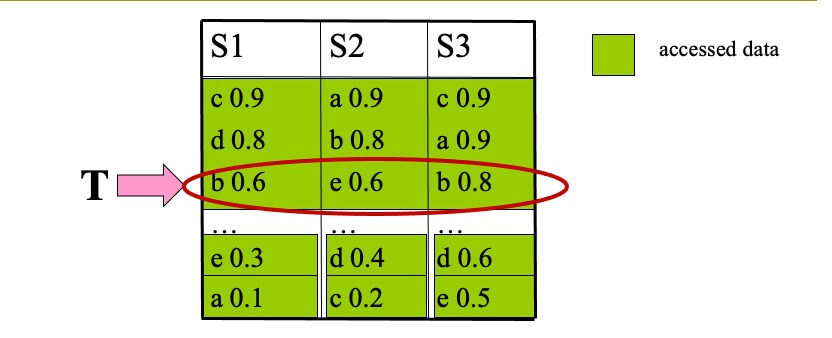#### Properties of TA

• Used as a standard module for merging ranked lists in many applications
• Usually finds the result quickly
• Depends on random accesses, which can be expensive
• random accesses are impossible in some cases
• e.g., an API allows to access objects incrementally by ranking score, but does not provide the score of a given object

### NRA: No Random Accesses

#### Introduction

• Iteratively retrieves objects and their atomic scores from the ranked inputs in a round-robin fashion.
• For each object x seen so far at any input maintain:
• f_x_ub: upper bound for x’s aggregate score (f_x)
• f_x_lb: lower bound for x’s aggregate score (f_x)
• W_k = k objects with the largest f^lb.
• If the smallest f^lb in W_k is at least the largest f_x_ub of any object x not in W_k, then terminate and report W_k as top-k result.

#### Example of NRA(k=1,f=sum)

• STEP 1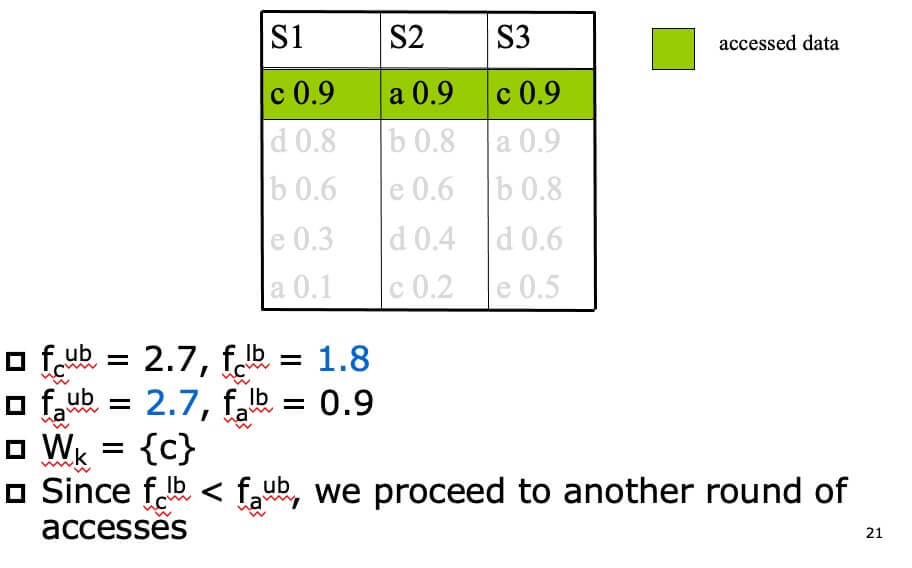• STEP 2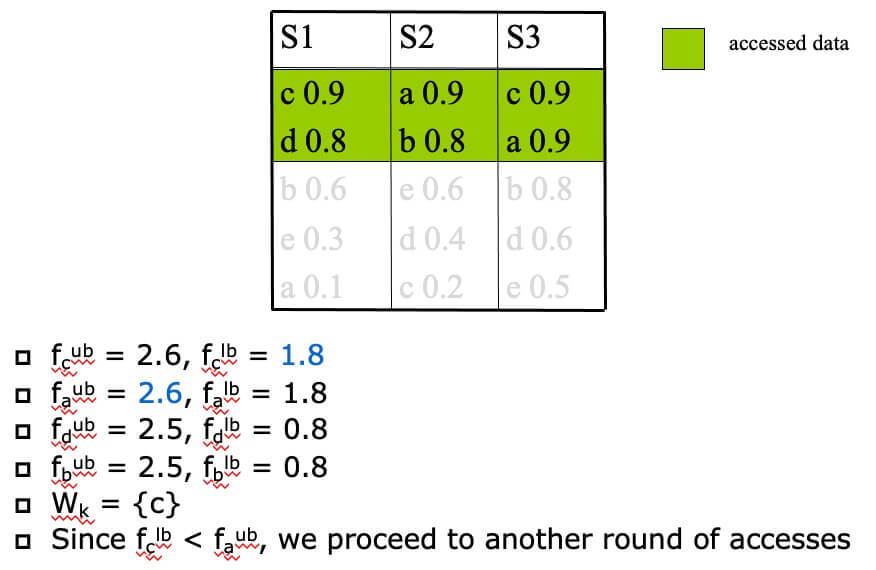• STEP 3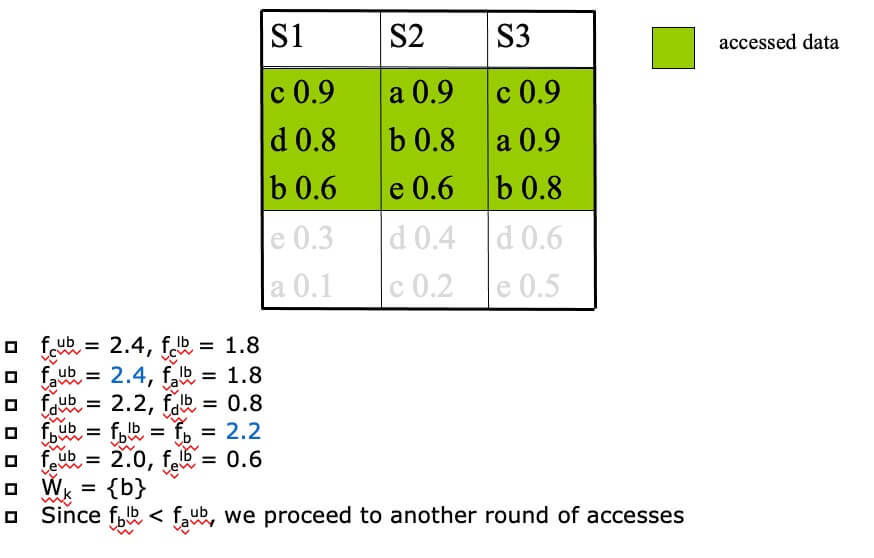• STEP 4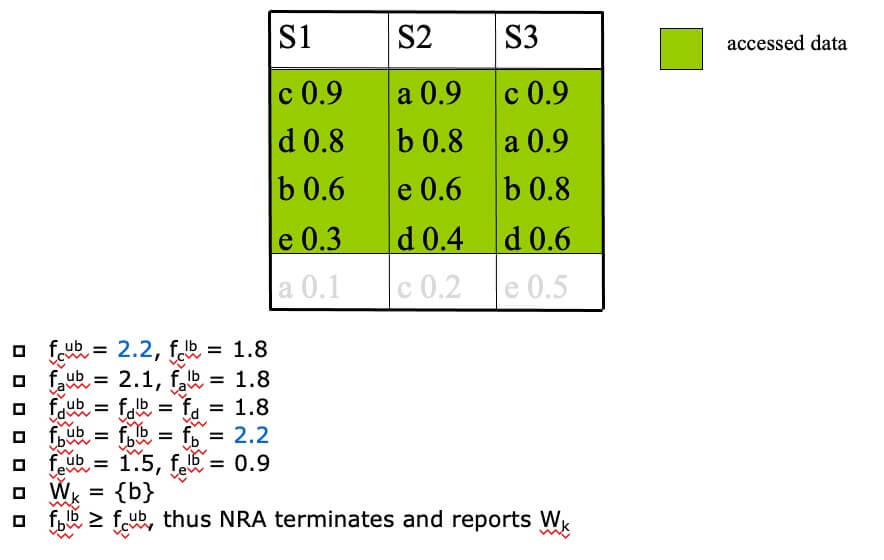#### NRA Properties

• More generic than TA, since it does not depend on random accesses
• Can be cheaper than TA, if random accesses are very expensive
• NRA accesses objects sequentially from all inputs and updates the upper bounds for all objects seen so far unconditionally.
• Cost: O(n) per access (the expected distinct number of objects accessed so far is O(n))
• No input list is pruned until the algorithm terminates

### LARA: LAttice-based Rank Aggregation

• LARA: An efficient NRA implementation
• Based on 3 observations about the top-k candidates
• Operates differently in the two (growing, shrinking) phases
• Takes its name from the lattice used in the shrinking phase
• Extendable to various top-k query variants

## 相关文章

2021-02-27
COMP7801 Topic 3 Spatial Networks
2021-02-06
COMP7801 Topic 2 Spatial Data Management
2021-01-30
COMP7801 Topic 1b Database Indexing
2021-01-23
COMP7801 Topic 1a Relational Database

Yu Zhang